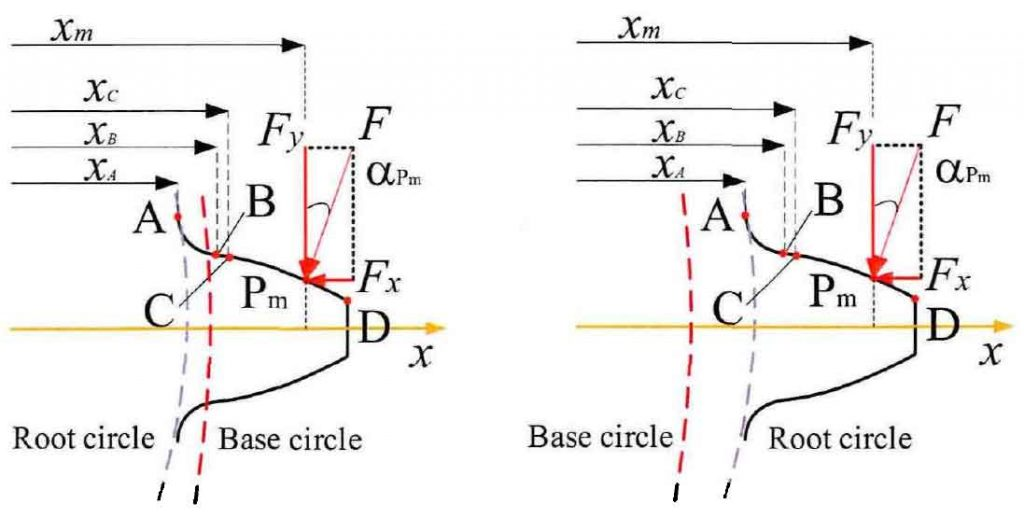# Simulation analysis of gear tooth profile(a) The base circle is larger than the root circle(b) The base circle is smaller than the root circle

The gear pair parameters are selected for tooth stiffness simulation analysis. The selected gear pair parameters cover two different tooth geometric forms shown in Figure 1. The numerical calculation method is used to calculate the tooth stiffness of the selected example parameters.